## Recent posts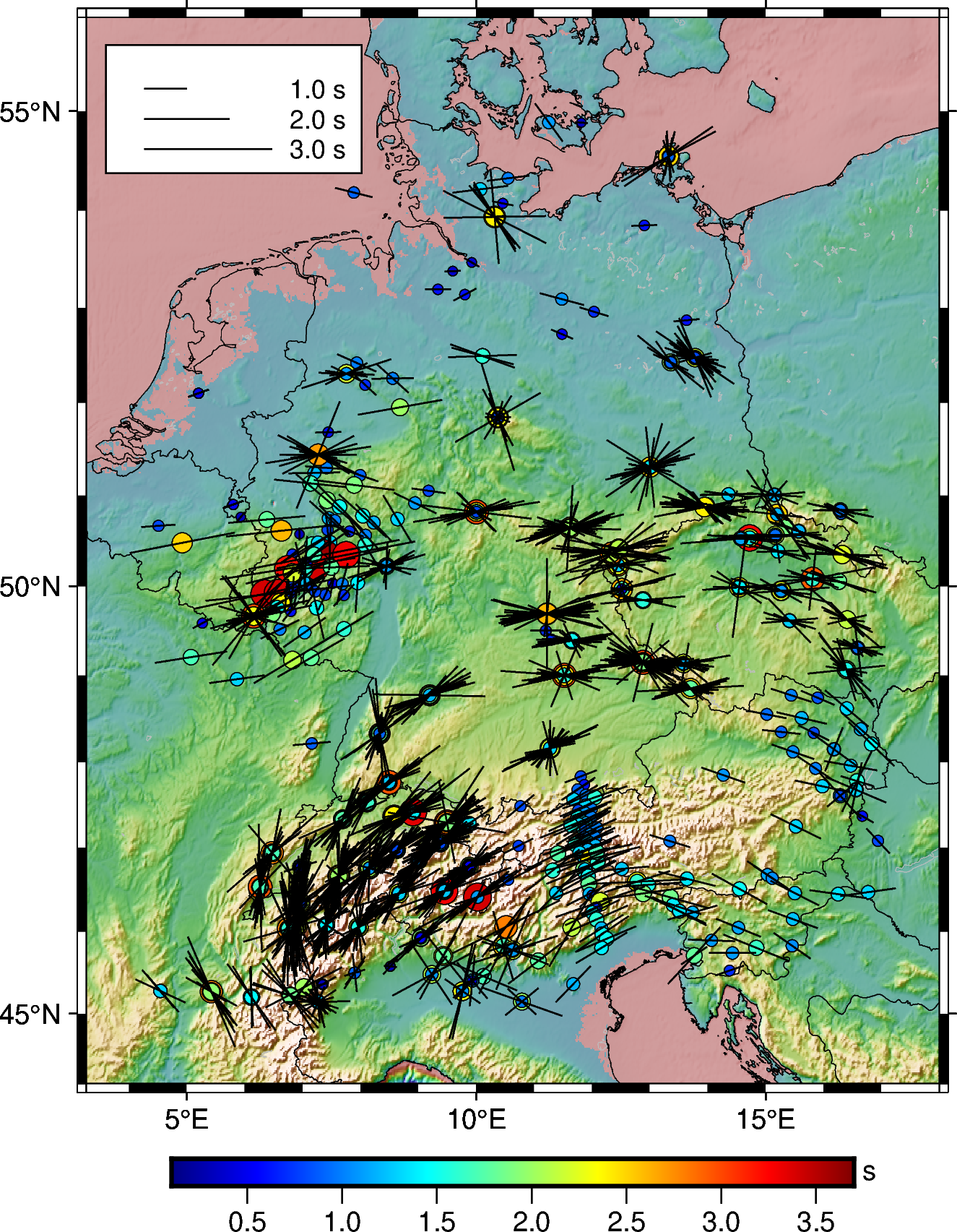#### How to plot shear-wave splitting measurements using pygmt

How you can plot the shear-wave splitting measurements from splitting database using PyGMT.How can you link unsplash images directly to your blog without hosting it locally.#### How to extract station data from the web page

Uses pandas to read the html page and extract the html data into pandas dataframe#### How to plot the earthquakes data on a topographic map

Read the earthquake data from a csv file and overlay on a topographic map using PyGMT.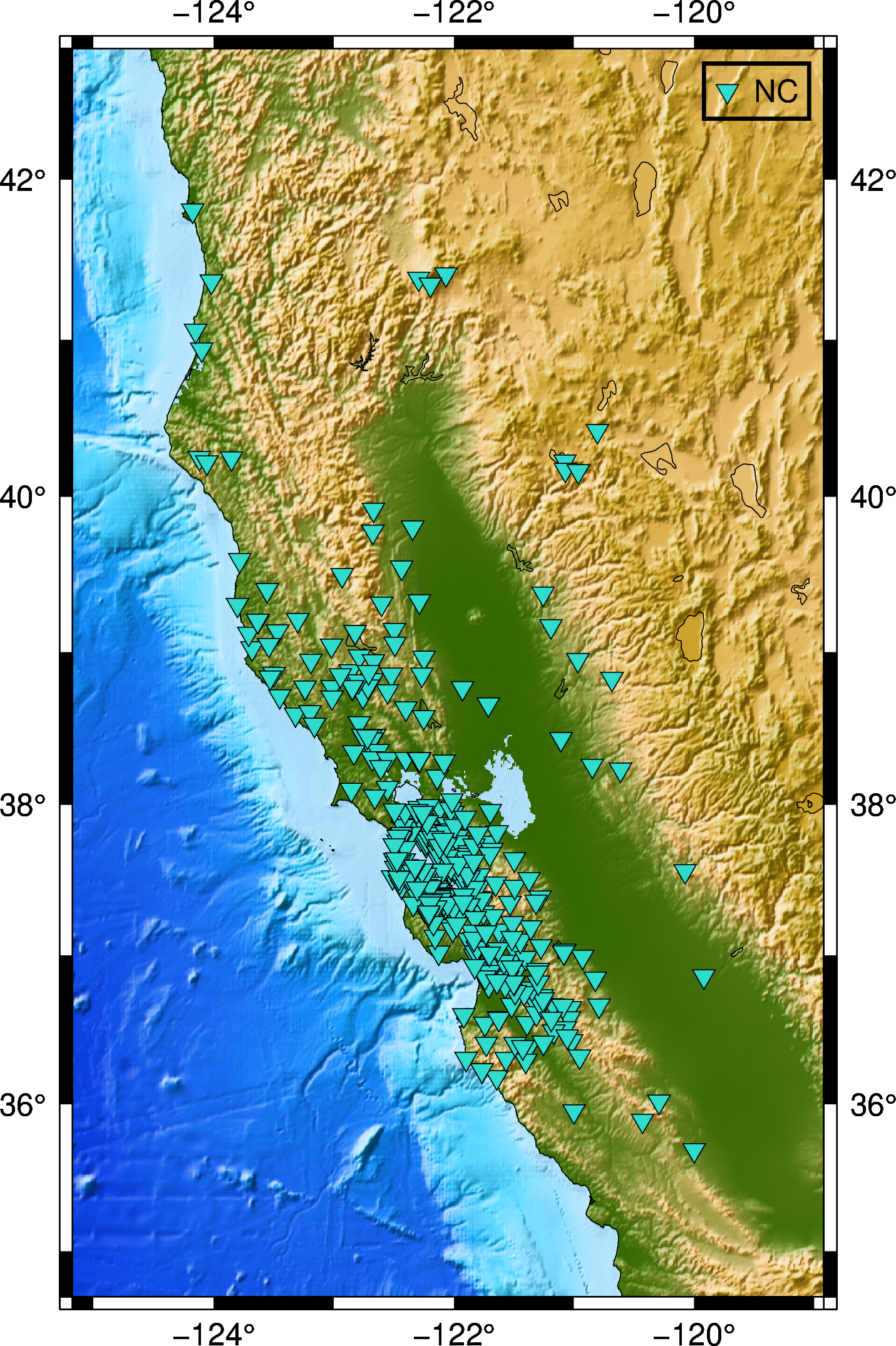#### How to automatically enquire the availability of seismic data using obspy

In this post, we will see how can we retrive the available seismic waveforms information for a given network, station, channel and client in a given period o...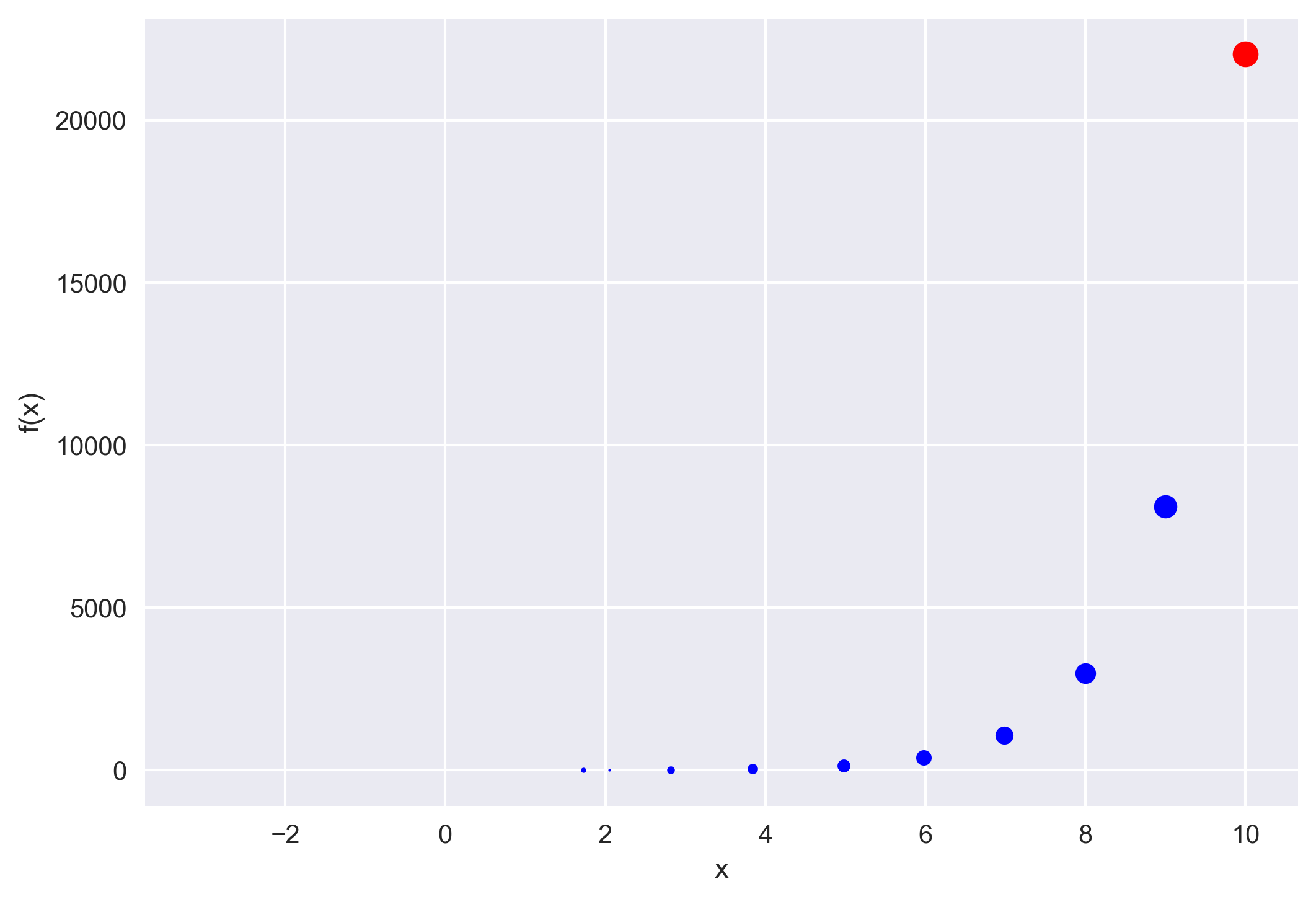#### How to implement the iterative newton–raphson method to find roots of a function in python

The Newton–Raphson method (commonly known as Newton’s method) is developed for finding roots of a given function or polynomial iteratively. We show two examp...#### How to host a http server on your linux computer to share files

Is the signal denoising using a wavelet effective? We will implement the wavelet-based signal denoising using the MATLAB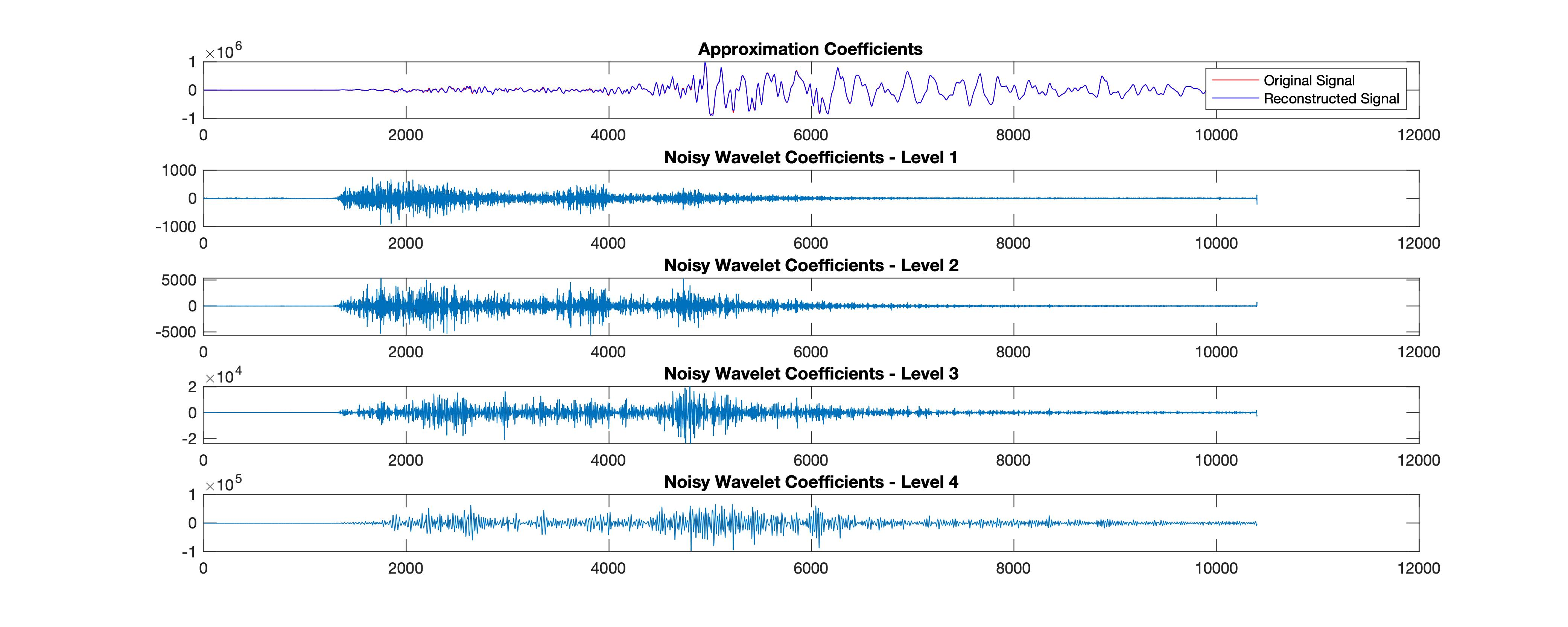#### How effective is the signal denoising using the matlab based wavelet analysis

We see how to download seismic waveforms, convert them into mat format from mini-seed and then perform denoising using wavelet analysis. We first performed w...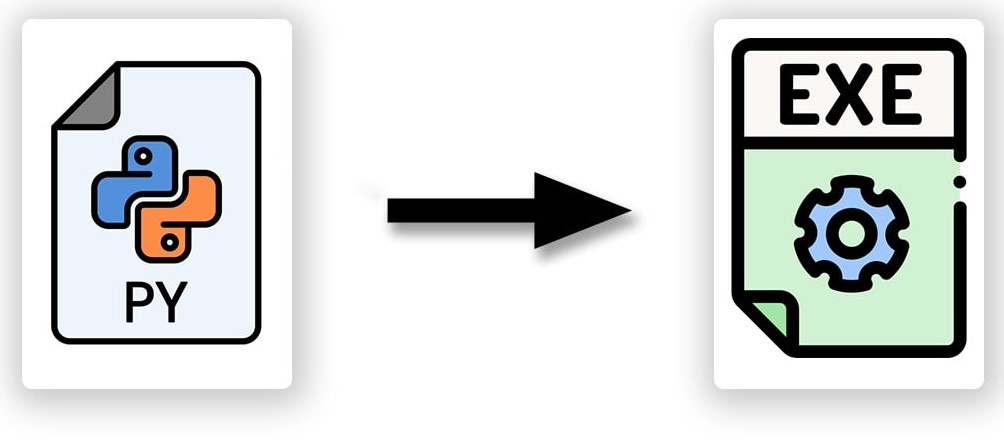#### Make your python script executable from anywhere in linux

Follow the instructions to make your python script executable from anywhere in Linux system.#### How to securely send data from local client to remote server using paramiko (codes included)

Paramiko module can be used in Python to securely send data from the local client to the remote server. It is analogous to the SSH and SCP in Linux.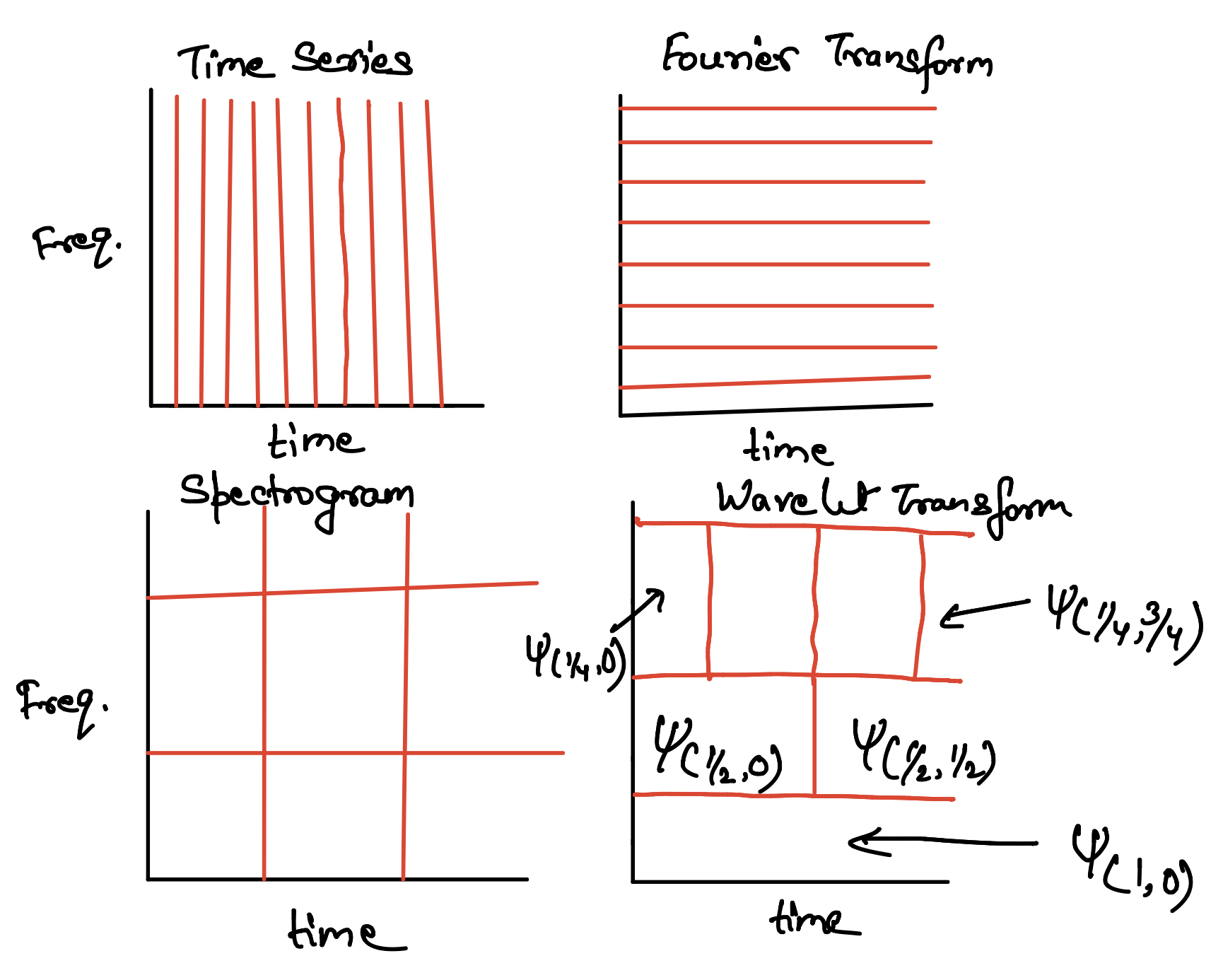#### Towards multi-resolution analysis with wavelet transform

We will learn the basic concepts of wavelet tranform and multi-resolution analysis starting from the Fourier Transform, and Gabor Transform.#### Empirical orthogonal function analysis to inspect the spatial coherency in the geospatial data (codes included)

Empirical Orthogonal Functions analysis decomposes the continuous space-time field into a set of orthogonal spatial patterns along with a set of associated u...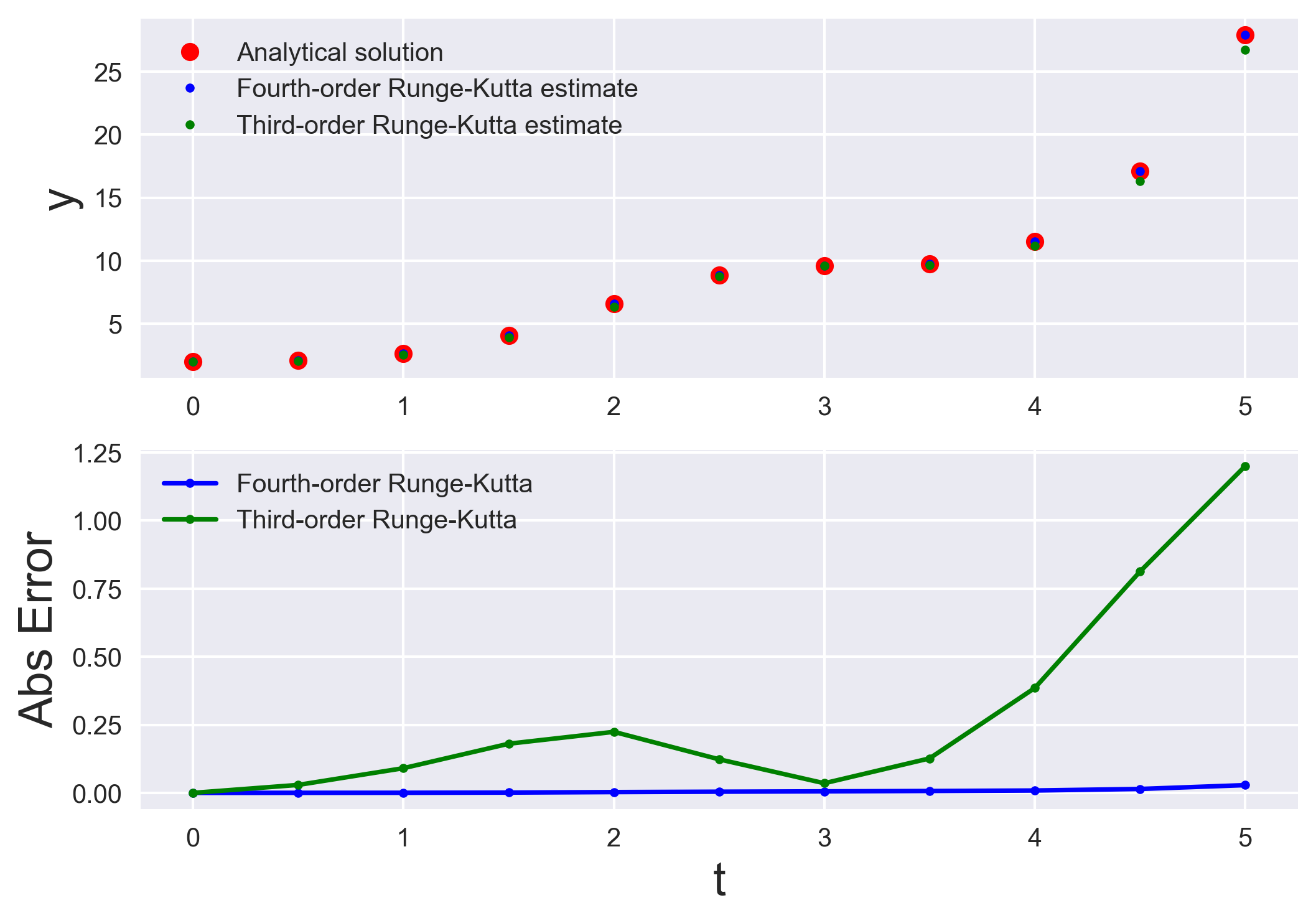#### Numerically solving initial value problems using the runge-kutta method

Runge-Kutta methods are most popular method to solve ordinary differential equations (ODEs) with a better approximation than the Euler method. We compare the...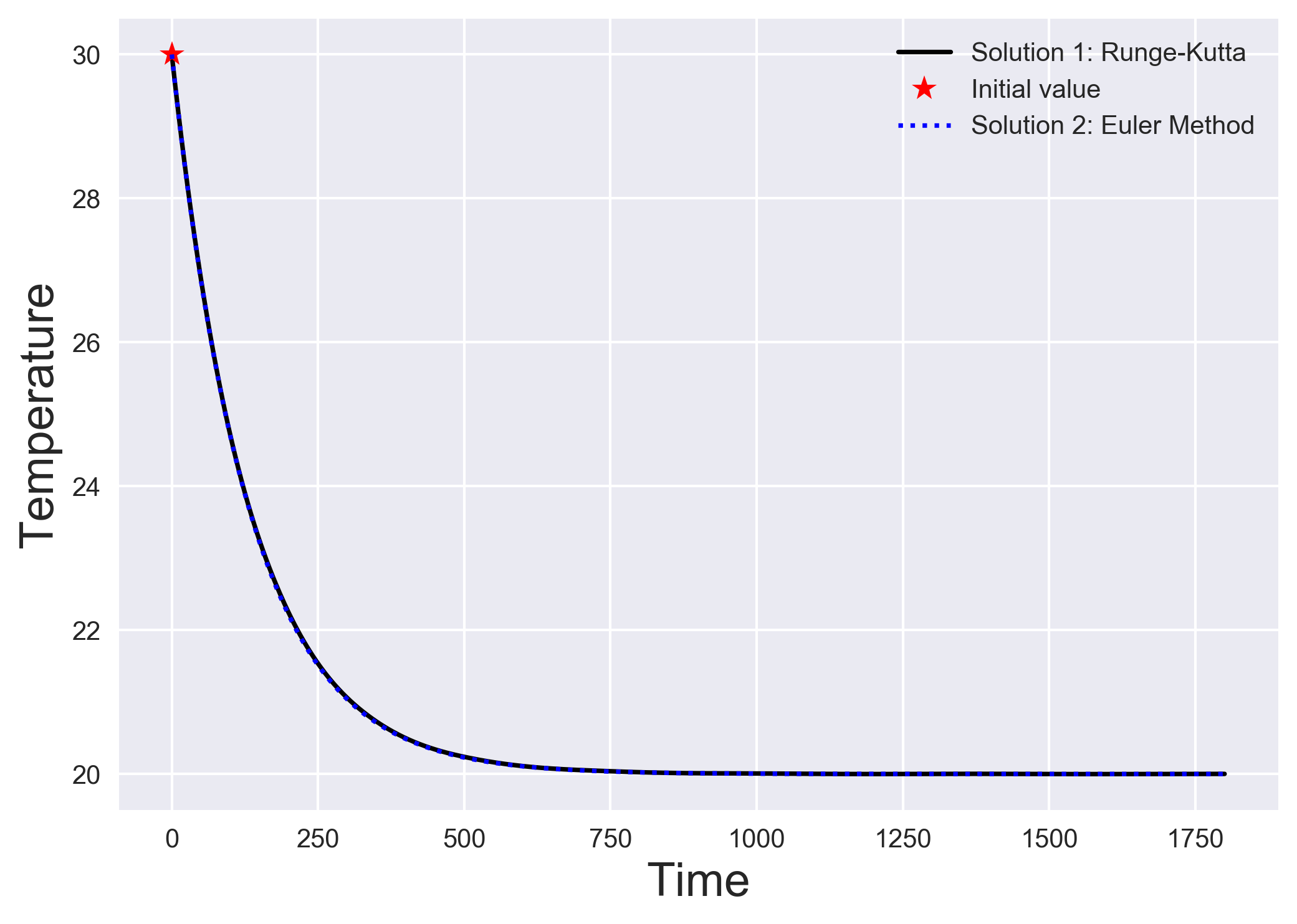#### Numerically solving initial value problems using the euler method

The simplest algorithm to solve a system of differential equations is the Euler method. We understand the Euler method by looking into a simple heat transfer...#### How to plot shear-wave splitting measurements using pygmt

How you can plot the shear-wave splitting measurements from splitting database using PyGMT.How can you link unsplash images directly to your blog without hosting it locally.#### How to extract station data from the web page

Uses pandas to read the html page and extract the html data into pandas dataframe#### How to plot the earthquakes data on a topographic map

Read the earthquake data from a csv file and overlay on a topographic map using PyGMT.#### How to automatically enquire the availability of seismic data using obspy

In this post, we will see how can we retrive the available seismic waveforms information for a given network, station, channel and client in a given period o...#### How to implement the iterative newton–raphson method to find roots of a function in python

The Newton–Raphson method (commonly known as Newton’s method) is developed for finding roots of a given function or polynomial iteratively. We show two examp...#### How to host a http server on your linux computer to share files

Is the signal denoising using a wavelet effective? We will implement the wavelet-based signal denoising using the MATLAB#### How effective is the signal denoising using the matlab based wavelet analysis

We see how to download seismic waveforms, convert them into mat format from mini-seed and then perform denoising using wavelet analysis. We first performed w...#### Make your python script executable from anywhere in linux

Follow the instructions to make your python script executable from anywhere in Linux system.#### How to securely send data from local client to remote server using paramiko (codes included)

Paramiko module can be used in Python to securely send data from the local client to the remote server. It is analogous to the SSH and SCP in Linux.#### Towards multi-resolution analysis with wavelet transform